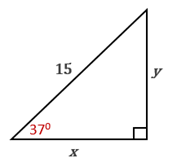Chapter 11.CT, Problem 8CTElementary Geometry For College St...

7th Edition
Alexander + 2 others
ISBN: 9781337614085

Solutions

Chapter
SectionElementary Geometry For College St...

7th Edition
Alexander + 2 others
ISBN: 9781337614085
Textbook Problem

In the drawing provided, find the value of y to the nearest whole number.To determine

To find:

The value of y to the nearest whole number.

Explanation

Procedure used:

In a right triangle, the sine ratio for an acute angle is the ratio oppsite sidehypotenuse.

Given:

The diagram as below

Calculation:

From the diagram, hypotenuse = 15, opposite side = y and the angle is 370.

Using the calculator, in the degree mode on entering 37 sin, we get the nearest value of sin 370 is 0.6018

Still sussing out bartleby?

Check out a sample textbook solution.

See a sample solution

The Solution to Your Study Problems

Bartleby provides explanations to thousands of textbook problems written by our experts, many with advanced degrees!

Get Started

Find more solutions based on key concepts